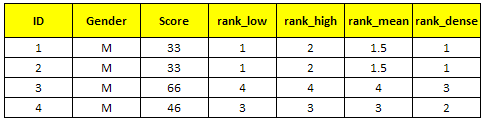# SAS : PROC RANK

This tutorial explains how to calculate rank for one or more numeric variables with PROC RANK. In SAS, there are multiple ways to calculate rank overall or by a grouping variable. In data step, it can be done via retain statement. SAS made it easy to compute rank with PROC RANK.

Create Sample Data
data temp;
input ID Gender \$ Score;
cards;
1 M 33
2 M 94
3 M 66
4 M 46
5 F 92
6 F 95
7 F 18
8 F 11
;
run;

Compute rank of numeric variable - "Score"
proc rank data= temp out = result;
var Score;
ranks ranking;
run;
Notes :
1. The OUT option is used to store output of the rank procedure.
2. The VAR option is used to specify numeric variable (s) for which you want to calculate rank
3. The RANKS option tells SAS to name the rank variable
4. By default, it calculates rank in ascending order.

Reverse order of ranking (Descending)

Suppose you need to assign the largest value of a variable as rank 1 and the last rank to the lowest value. The descending keyword tells SAS to sort the data in descending order and assign rank to the variable accordingly.
proc rank data= temp descending out = result;
var Score;
ranks ranking;
run;
Percentile Ranking (Quartile Rank)

Suppose you need to split the variable into four parts, you can use the groups option in PROC RANK. It means you are telling SAS to assign only 4 ranks to a variable.
proc rank data= temp descending groups = 4 out = result;
var Score;
ranks ranking;
run;
Note :
GROUPS=4 for quartile ranks, and GROUPS=10 for decile ranks, GROUPS = 100 for percentile ranks.

Ranking within BY group (Gender)

Suppose you need to calculate rank by a grouping variable. To accomplish this task, you can use the by statement in proc rank. It is required to sort the data before using by statement.
proc sort data = temp;
by gender;
run;
proc rank data= temp descending out = result;
var Score;
ranks ranking;
by Gender;
run;

How to compute rank for same values

Let's create a sample dataset. See the variable score having same values (33 appearing twice).
data temp2;
input ID Gender \$ Score;
cards;
1 M 33
2 M 33
3 M 66
4 M 46
;
run;
Specify option TIES = HIGH | LOW | MEAN | DENSE in PROC RANK.
proc rank data= temp2 ties = dense out = result;
var Score;
ranks rank_dense;
run;
1. LOW - assigns the smallest of the corresponding ranks.
2. HIGH - assigns the largest of the corresponding ranks.
3. MEAN - assigns the mean of the corresponding ranks (Default Option).
4. DENSE - assigns the smallest of the corresponding rank and add +1 to the next rank (don't break sequence)
See the comparison between these options in the image below -SAS : Handle Ties in PROC RANKShare
Related PostsDeepanshu founded ListenData with a simple objective - Make analytics easy to understand and follow. He has over 10 years of experience in data science. During his tenure, he has worked with global clients in various domains like Banking, Insurance, Private Equity, Telecom and Human Resource.

11 Responses to "SAS : PROC RANK"
1.super good..keep going..

2.very good and easy explanation....

3.I didn't get the concept of ties that you have explained in the last!

1.Thank you for your feedback. I have added more description to the concept of ties. Hope it helps.

4.Nice explanation... keep going guys.. Thanks!!

5.This comment has been removed by the author.

6.Nice explanation..

7.How can we get rank for character variables

8.How can I get the cuts of deciles along with ranks and frequency as provided by proc rank?

9.Can you please the proc rank concept along with proc sql?

10.gracias, muy buena explicación

Next → ← Prev